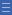## Featured resource

Home > Student activities > National Maths Day > National Maths Day 2015 > Activity: Triangle midpoints

# Activity: Triangle midpoints

Activity: Triangle midpoints

Construct a triangle from its three midpoints.

## Find the activity on NRICH

Go to http://nrich.maths.org/483 (link will open in a new window)

## More questions

What is the relationship between the original triangle and the triangle formed by joining the three midpoints? Is it always possible to draw a triangle given three points that are supposed to be the midpoints of its sides?

Given four points that are the midpoints of the sides of a quadrilateral, how would you draw the original quadrilateral? Is it possible for any set of four points?

## Shine a light on the Proficiencies

A useful practice to encourage problem solving is to reverse the question. This question reverses the more standard question of the relationship between the medians of a triangle and the sides of the triangle.

Asking “How many ways?” shows that mathematical problems often have more than answer. An important question to ask is how we know we have found all the possible answers, which highlights the importance of reasoning. In this case there is a relatively simple proof that there is only one way to draw the triangle.

Asking always/sometimes/never questions (such as “Is it always possible?”) encourages students to employ mathematical reasoning to consider special cases, or to prove that it is always or never possible. The quadrilateral question is a good example of where there needs to be a special arrangement of points in order to draw the quadrilateral. It leads to a relatively little known but delightful and easy to prove result about the quadrilateral formed by joining the midpoints of the sides of any quadrilateral. Deductive proof is a cornerstone of mathematical reasoning.

Yes

Yes

Name Class Section PriorityDownload Triangle Midpoints (blank worksheet) File 1